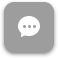Call Now 855-300-1469

# BUS 5421 Managerial Economics

#### Course Description

Provides an understanding of the microeconomic forces that influence firm decision making. Includes competitive markets and market failure, benefit-cost analysis, demand estimation and forecasting, decision making under risk and uncertainty, production and cost estimation, and market structure analysis.

#### Course Objectives

The objective of this course is to help business students become more adept at designing and developing business strategy at the firm level. Students should gain a rigorous understanding of competitive markets as well as alternative market structures such as monopoly, oligopoly, and monopolistic competition. Engaged students should also acquire a basic competence in the theoretical derivation and empirical estimation of demand, cost, and production functions, answering the fundamental managerial questions of what, how, and for whom to produce.

### Week 1

##### Lecture: Introduction

Outcomes

• Describe the rational-actor paradigm, and use the paradigm to predict behavior
• List and explain the two main reasons why mistakes are made by decision makers
• Define the terms wealth, value and willingness to pay
• Explain how voluntary transactions create wealth
• Describe how tax, subsidy and price control policies can destroy wealth
• Define and illustrate the concepts of fixed costs, variable costs and opportunity costs
• Define and give examples of the fixed-cost and hidden-cost fallacies

### Week 2

##### Lecture: Making Extent Decisions: Background Materials

Outcomes

• Explain the difference between average and marginal costs, and describe how marginal analysis is used to make extent decisions
• Calculate MC and make profitable decisions using the marginal analysis rule
• Describe some problems when moving from theory to practice, and how best to deal with these problems
• Identify the relevant benefits and costs of entry and shutdown decisions
• Calculate break-even quantity and break-even price, and use these concepts to make profitable entry and shutdown decisions
• Distinguish fixed costs from sunk costs
• Identify two investments that are relationship specific
• Define the terms sunk cost and post-investment hold-up
• Describe two strategies that reduce transaction costs and encourage relationship specific investments

### Week 3

##### Lecture: Stay-Even Analysis

Outcomes

• Explain how marginal analysis is used to find the profit maximizing price
• Calculate and interpret the price elasticity demand
• Use price elasticity information to calculate marginal revenue, and to make profitable simple pricing decisions
• Calculate a table of stay-even quantities and construct a stay-even curve
• Make profitable pricing decisions by comparing the stay-even curve with the demand curve
• Describe the relationship between marginal productivity and marginal costs
• Explain why cost curves are U-shaped
• Identify three types of long-run scale economies
• Define the term economies of scope, and provide examples of how consolidation can produce economies of scope
• Describe strategies that a single product company can use to benefit from the economies of scope of others

### Week 4

##### Lecture: Demand and Supply: Value and Surplus

Outcomes

• Understand and explain the concepts of value and surplus for both consumers and producers
• Explain why demand curves slope downward and supply curves slope upward
• Predict the impact on equilibrium price and quantity of a change in demand or supply
• Define a market in terms of product, time and geographic dimension
• Outline the analytical steps in correctly using the demand/supply model
• Explain the concept of mean reversion, and explain why firm profits revert to an average rate of return in competitive industries
• Explain why, despite inherent competitive advantages, monopoly profits eventually erode as well
• List and distinguish between industry-based and resource-based determinants of long-run profitability
• Describe the various strategies that can be used to gain or maintain a sustainable competitive advantage

### Week 5

##### Lecture: Pricing Commonly Owned Products

Outcomes

• Describe pricing strategies for jointly owned substitutes and jointly owned complements
• Explain how capacity decisions are made, and how pricing decisions are made after capacity is built
• Explain the effect advertising expenditures have on demand elasticity for both price-related promotions and product-related promotion, and how to price in each situation
• List the conditions needed for direct price discrimination
• Explain how to price to groups with different elasticity
• Describe implementation strategies for direct price discrimination
• Provide examples of indirect price discrimination and list its primary disadvantages
• Develop pricing strategies that avoid cannibalizing sales from a high margin product

### Week 6

##### Lecture: The Theory Behind Game Theory

Outcomes

• Define Nash equilibrium
• Find the Nash equilibrium in a sequential move game
• Explain how credible threats can change the outcomes in game theory
• Find the Nash equilibrium of the Prisoner’s Dilemma
• Explain the tension between conflict and cooperation
• List strategies to avoid or get out of games that are like the Prisoner’s Dilemma
• Explain how a player can gain an advantage by shifting a simultaneous move game to a sequential game; and how the first-move advantage can be negated by a credible threat
• Describe the non-strategic view of bargaining and based on this view, list strategies that can increase bargaining power

### Week 7

##### Lecture: Moral Hazard

Outcomes

• Calculate expected benefits and costs
• List the three benefits of modeling uncertainty
• Use a decision tree to model uncertainty
• List and describe the main attributes of each auction type and the inherent tradeoffs of each
• Explain the consequences of adverse selection, and provide examples of adverse selection and how to solve it
• Describe the moral hazard problem
• Distinguish adverse selection from moral hazard
• Explain behavior using both adverse selection and moral hazard explanations
• Calculate break-even values to induce hard work and negate shirking

### Week 8

##### Lecture: Aligning Employee Incentives with Firm Goals

Outcomes

• Explain the principle-agent problem and how it relates to incentive conflicts
• Describe strategies to resolve problems that arise due to the principle-agent relationship